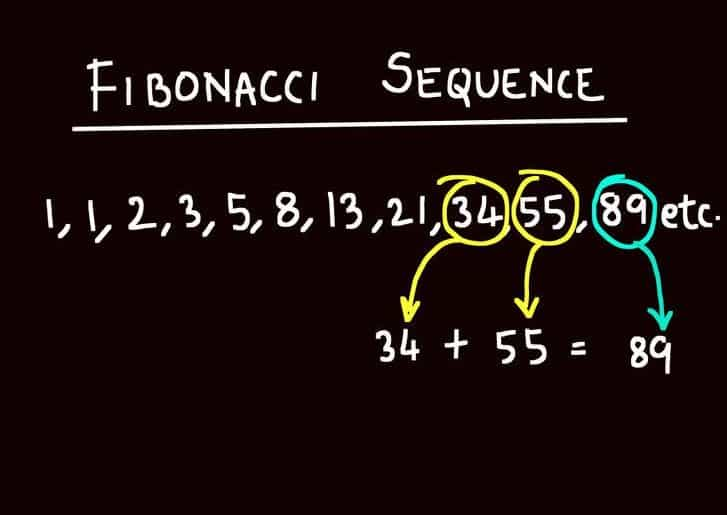What is Fibonacci RetracementWhat is Fibonacci Retracement
April 14, 2016

Step 1: Understanding the Fibonacci Sequence

As Italians named Leonardo go, Fibonacci is, at most, the second most famous (some guy named Da Vinci takes the number one spot). However, Fibonacci can do something for you that Da Vinci never could, fibonacci can make you money. Fibonacci’s fascination with numbers led him to discover the mathematical sequence that bears his name (also known as the Golden Ratio).

The Fibonacci sequence is as follows: 1, 1, 2, 3, 5, 8, 13, 21, 34, 55, 89, 144, 233, 377… You may have seen this sequence of numbers on a test, a puzzle or in popular fiction like The DaVinci Code. But what does this sequence actually mean?

Fibonacci’s sequence says that, in a group of three numbers, the sum of the first two numbers will always equal the next number in the sequence.

1 + 1 = 2
1 + 2 = 3
2 + 3 = 5
3 + 5 = 8

You can see how the pattern works. Take whatever number the equation totals, add it to the second number in the equation and the new total will equal the next number in the sequence.

Step 2: Finding Fibonacci’s Golden Ratio & More

Now that you know how his sequence works, you can learn how to find what traders call “the golden mean” or “golden ratio”. You arrive at the golden mean by taking any number in the sequence and dividing it by the number that immediately follows it. This answer will be .61 or 61% (or thereabouts). So if we bring up our sequence again…..

1, 1, 2, 3, 5, 8, 13, 21, 34, 55, 89, 144, 233, 377

…you can take:

13 ÷ 21 and get 0.619
21 ÷ 34 and get 0.617
34 ÷ 55 and get 0.618

You can use the sequence to get other important ratios as well. These numbers are: 0.38 (dividing any number by the one two places to its right), 0.50 (the sum of three numbers divided by two), .786 (the square root of .61), 1.618 (dividing by the number that immediately precedes your number), and 1.27 (the square root of 1.618).

The reason why Fibonacci’s sequence is such a big deal is because it can be found all around nature. Everything from seashells and starfish, to trees and the distance between your elbow and hand all follow the patterns first discovered by Fibonacci. And there is strong evidence that patterns in the Forex follow this sequence as well.

Based on your entry point in the market, you can place lines on your chart that correspond to the numbers laid out by the various equations listed above (.61, .38, .78 etc.). It has been shown that, over time, the market’s rises and falls generally match up with Fibonacci’s lines. In theory, if you set your buy and sell points according to these patterns, you will be able to sell before the market turns down, and buy before it goes back up.

Finding the Fibonacci numbers are difficult enough, putting them on your chart is even more difficult, and fully understanding how they work is the most difficult of all.  There’s no reason why you should try and understand this yourself.SummaryArticle Name
Description
It has been shown that, over time, the market’s rises and falls generally match up with Fibonacci’s lines. In theory, if you set your buy and sell points according to these patterns, you will be able to sell before the market turns down, and buy before it goes back up.
Author
Publisher Name